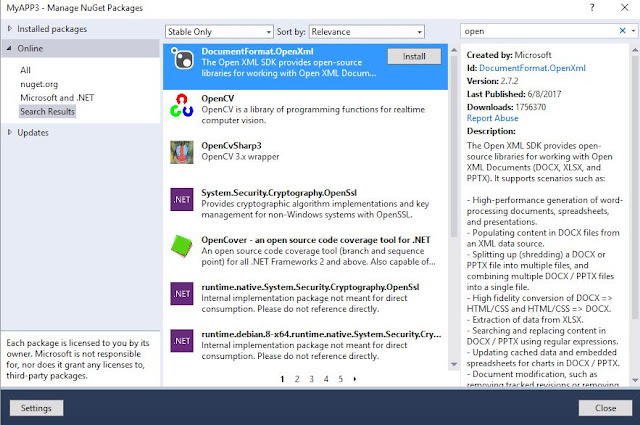Simplest way to convert some large Datasets to Excel files is using OpenXML and Closed XML, this can be used for console application as well as Web applications. To create the Excel files (.XLSX) we need to Add the Open XML and Closed XML reference from nugget packages as below.OPENXMLClosed XML

Here am converting Dataset into Excel file, if data set contains multiple DataTables, it can be looped into same Excel with different sheet. Below is the sample code to connect Generate Excel(.xlsx) and save in some application path.
To use the OpenXML and Closed XML we need to use below namespaces

using ClosedXML.Excel

Sample Code :

using System;
using System.Collections.Generic;
using System.Linq;
using System.Text;
using ClosedXML.Excel;
using System.Data;

namespace MyAPP3
{
class Program
{
static void Main(string[] args)
{
DataSet ds = new DataSet();
DataTable dt = new DataTable();
ExportDataSetToExcel(ds);
}

public static void  ExportDataSetToExcel(DataSet ds)
{
string AppLocation = "";
AppLocation = System.IO.Path.GetDirectoryName(System.Reflection.Assembly.GetExecutingAssembly().CodeBase);
AppLocation = AppLocation.Replace("file:\\", "");
string date = DateTime.Now.ToShortDateString();
date = date.Replace("/", "_");
string filepath = AppLocation + "\\ExcelFiles\\" + "RECEIPTS_COMPARISON_" + date + ".xlsx";

using (XLWorkbook wb = new XLWorkbook())
{
for (int i = 0; i < ds.Tables.Count; i++)
{